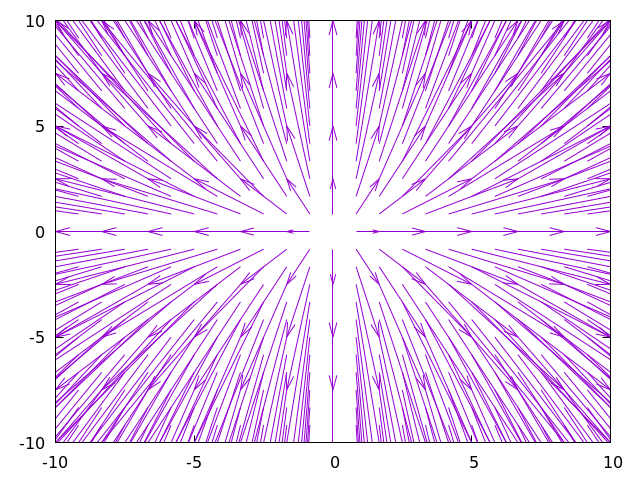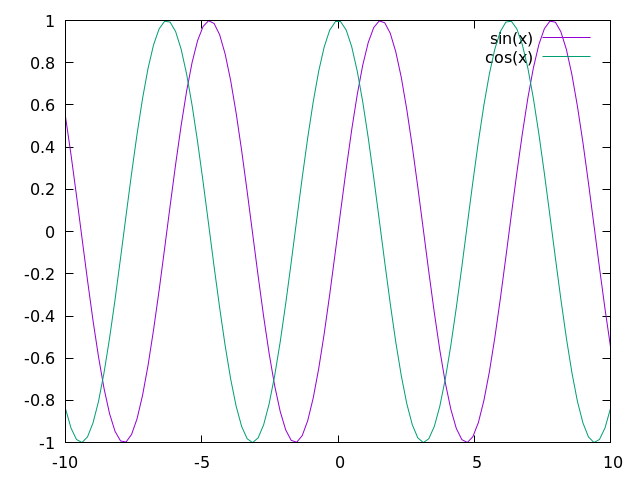# Plotting with gnuplot

The common-doc-gnuplot system allows you to render plots using gnuplot commands from inside the document.

## API

gnuplot
gnuplot plot.
• path
Path to file where the image will be stored.

## Examples

Rendering a direction field:

@begin[path=dir-field.png](gnuplot)
@begin(verb)
set xrange [-10:10]
set yrange [-10:10]
set samples 25
set isosamples 25
set key off
dx(x) = x
dy(x) = 2*x
plot "++" using 1:2:(dx($1)):(dy($2)) w vec
@end(verb)
@end(gnuplot)The sine and cosine functions:

@begin[path=sin-cos.png](gnuplot)
@begin(verb)
plot sin(x), cos(x)
@end(verb)
@end(gnuplot)# Include Files

The common-doc-include contrib loads external text files into the document. The path attribute is the path to the file, and the optional start and end attributes can be used to specify a numeric range of lines.

## API

include
Include an external file.
• path
Path to the local file to include.
• start
The line where the inclusion will start.
• end
The line where the inclusion will end.

## Examples

The source code of this documentation uses common-doc-include extensively.

# Split Paragraphs

The common-doc-split-paragraphs contrib splits text nodes on double newlines into separate paragraphs.

## API

*paragraph-separator-regex*
A regular expression that matches double newlines.
split-paragraphs(node)
Recursively go through a document, splitting paragraphs in text nodes into paragraph nodes.

## Examples

(defpackage split-paragraphs-example
(:use :cl :common-doc)
(:import-from :common-doc.split-paragraphs
:split-paragraphs))
(in-package :split-paragraphs-example)

(defvar *document* (make-content
(list
(make-text
(format nil "Some text.~%~%Some "))
(make-bold
(list (make-text "bold text")))
(make-text (format nil ".~%~%Other text.")))))

(dump *document*)

;; content-node
;;   text-node
;;     "Some text.
;;
;; Some "
;;   bold
;;     text-node
;;       "bold text"
;;   text-node
;;     ".
;;
;; Other text."

(setf *document* (split-paragraphs *document*))

(dump *document*)

;; content-node
;;   paragraph
;;     text-node
;;       "Some text."
;;   paragraph
;;     text-node
;;       "Some "
;;     bold
;;       text-node
;;         "bold text"
;;     text-node
;;       "."
;;   paragraph
;;     text-node
;;       "Other text."


# TeX

The common-doc-tex contrib is just a couple of macros that expand to TeX notation. They are useful for two reasons:

1. Using them is more semantic than using the resulting $...$ syntax.
2. The macroexpansion can be overriden.

## API

tex
Inline TeX code.
tex-block
Block of TeX code.

## Examples

The following Scriba input:

The derivative of a function @tex(f) at @tex(a), @tex(f'(a)), is

@begin(texb)
f'(a) = \lim_{h \to 0} \frac{f(a+h)-f(a)}{h}
@end(texb)

Expands to the following:

The derivative of a function $f$ at $a$, $f'(a)$, is

$$f'(a) = \lim_{h \to 0} \frac{f(a+h)-f(a)}{h}$$